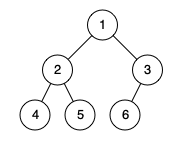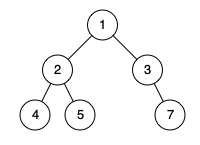# 958. Check Completeness of a Binary Tree ⭐

Medium
Given the `root` of a binary tree, determine if it is a complete binary tree.
In a complete binary tree, every level, except possibly the last, is completely filled, and all nodes in the last level are as far left as possible. It can have between `1` and `2h` nodes inclusive at the last level `h`.
Example 1:Input: root = [1,2,3,4,5,6]
Output: true
Explanation: Every level before the last is full (ie. levels with node-values {1} and {2, 3}), and all nodes in the last level ({4, 5, 6}) are as far left as possible.
Example 2:Input: root = [1,2,3,4,5,null,7]
Output: false
Explanation: The node with value 7 isn't as far left as possible.
Constraints:
• The number of nodes in the tree is in the range `[1, 100]`.
• `1 <= Node.val <= 1000`

### 解題

Runtime: 0 ms, faster than 100%
Memory Usage: 3 MB, less than 100%
/**
* Definition for a binary tree node.
* type TreeNode struct {
* Val int
* Left *TreeNode
* Right *TreeNode
* }
*/
func isCompleteTree(root *TreeNode) bool {
if root == nil {
return true
}
queue := make([]*TreeNode, 0)
queue = append(queue, root)
flag := false
for len(queue) > 0 {
top := queue
queue = queue[1:]
if top.Left != nil {
if flag { return false }
queue = append(queue, top.Left)
} else {
flag = true
}
if top.Right != nil {
if flag { return false }
queue = append(queue, top.Right)
} else {
flag = true
}
}
return true
}